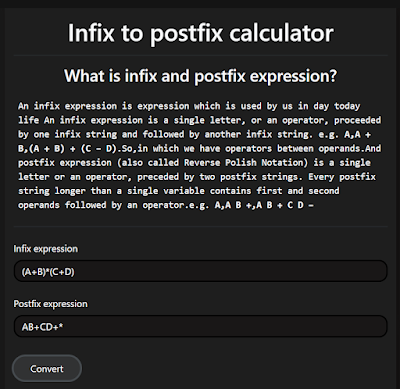# Step-by-Step Guide: Building an Infix to Postfix Converter with JavaScript & HTML

Ever wanted to add a powerful calculator feature to your website? You're not alone. Many web developers recognize the importance of mathematical operations for various online tools. This step-by-step guide will walk you through creating an infix to postfix converter using JavaScript and HTML. It's a key component for building effective calculators and more. Let’s dive in and get started

###Photo by Towfiqu barbhuiya on UnsplashWhat is a Infix to Postfix Converter?

An infix-to-postfix converter is a tool or algorithm that transforms expressions written in infix notation (where the operator is between the operands) to postfix notation (where the operator follows the operands). This conversion is essential because computers can process postfix expressions more efficiently, without the need for parentheses to determine the order of operations. For example, the infix expression "A + B" gets converted to "A B +". Using such a converter makes evaluating complex mathematical expressions simpler and faster for machines.

### Building the Converter

To build a infix to postfix converter, we'll use HTML, CSS, and JavaScript. Here's the HTML code for our calculator form:

``````<form>
<div class="form-group my-4">
<label>Infix Expression</label>
<input class="form-control" id="Infix" required="" type="text" />
</div>
<div class="form-group">
<label>Postfix Result</label>
<input class="form-control" disabled="" id="PostfixResult" type="text" />
</div>
<button class="btn btn-outline-secondary mb-3 text-light convert" type="submit">
Convert
</button>
</form>
``````

This design includes an input field for the infix expression and a field to display the postfix result. We've used Bootstrap classes to style the form.

Next, it's time to write the JavaScript code to convert the infix expression to convert into postfix expression. You can write this JavaScript in script tag within same html file or can create different js file & link that js in html file.

Here is the full code:

``````document.querySelector('.convert').addEventListener('click', function(e) {
e.preventDefault();
var infixed = document.getElementById('Infix').value;
var infix = infixed.split("");
var stack = [], postfix = [];

function isOperand(operand) {
return ((operand >= 'a' && operand <= 'z') ||
(operand >= 'A' && operand <= 'Z') ||
(operand >= '0' && operand <= '9'));
}

function precedence(prec) {
switch(prec) {
case '(': return 4;
case '^':
case '%': return 3;
case '*':
case '/': return 2;
case '+':
case '-': return 1;
case ')': return 0;
default: return -1;
}
}

for (var i = 0; i < infix.length; i++) {
if (isOperand(infix[i])) {
postfix.push(infix[i]);
} else if (stack.length == 0) {
stack.push(infix[i]);
} else if (infix[i] == ')') {
while (stack[stack.length - 1] != '(') {
postfix.push(stack.pop());
}
stack.pop();
} else if (precedence(infix[i]) > precedence(stack[stack.length - 1])) {
stack.push(infix[i]);
} else {
while (precedence(infix[i]) <= precedence(stack[stack.length - 1]) && stack.length != 0 && stack[stack.length - 1] != '(') {
postfix.push(stack.pop());
}
stack.push(infix[i]);
}
}
while (stack.length != 0) {
postfix.push(stack.pop());
}
var strPost = postfix.join('');
document.getElementById("PostfixResult").value = strPost;
});``````

And here it's step by step explanation with code snippets:

1. Start by adding a click event listener to our "Convert" button, which will trigger the conversion when clicked.:
2. ``````document.querySelector('.convert').addEventListener('click', function(e) {
// code goes here
});``````
3. Get the user's infix expression input. Additionally, initialize arrays: one for the stack to hold operators and another to hold the postfix output.
4. ``````var infixed = document.getElementById('Infix').value;
var infix = infixed.split("");
var stack = [], postfix = [];
``````
5. Create a helper function to check if the given character is an operand. In our case, it checks for letters and numbers.
6. ``````function isOperand(operand) {
return (operand >= 'a' && operand <= 'z') || (operand >= 'A' && operand <= 'Z') || (operand >= '0' && operand <= '9');
}
``````
7. Another helper function sets the precedence for operators. This ensures that operators like multiplication and division are processed before addition and subtraction.
8. ``````function precedence(prec) {
switch(prec) {
case '(': return 4;
case '^':
case '%': return 3;
case '*':
case '/': return 2;
case '+':
case '-': return 1;
case ')': return 0;
default: return -1;
}
}
``````
9. Loop through each character of the infix expression:
• If it's an operand, add it directly to the postfix array.
• If the stack is empty or the character is an open parenthesis, push it onto the stack.
• If it's a close parenthesis, pop operators off the stack until an open parenthesis is encountered.
• Otherwise, while the precedence of the current operator is less than or equal to the top of the stack, pop the stack and append to postfix. After this, push the current operator onto the stack.
``````for (var i = 0; i < infix.length; i++) {
if (isOperand(infix[i])) {
postfix.push(infix[i]);
} else if (stack.length == 0) {
stack.push(infix[i]);
} else if (infix[i] == ')') {
while (stack[stack.length - 1] != '(') {
postfix.push(stack.pop());
}
stack.pop();
} else if (precedence(infix[i]) > precedence(stack[stack.length - 1])) {
stack.push(infix[i]);
} else {
while (precedence(infix[i]) <= precedence(stack[stack.length - 1]) && stack.length != 0 && stack[stack.length - 1] != '(') {
postfix.push(stack.pop());
}
stack.push(infix[i]);
}
}``````
10. After processing the entire infix string, pop any remaining operators from the stack and append them to the postfix array.
``````while (stack.length != 0) {
postfix.push(stack.pop());
}``````
11. Convert the postfix array to a string and set it as the value for the result input field. Additionally, prevent the form's default submission behavior to avoid reloading the page.
12. ``````var strPost = postfix.join('');
document.getElementById("PostfixResult").value = strPost;
e.preventDefault();``````
Here's how the converter looks after some CSS modifications and using built-in Bootstrap classes. You can see a screenshot of the updated design below and test it using this link.calcont

You can checkout how I developed this converter in calcont's github repo & can contribute to it as well.

Hope you got the idea for developing Infix to Postfix converter using html & javascript, Thanx !!